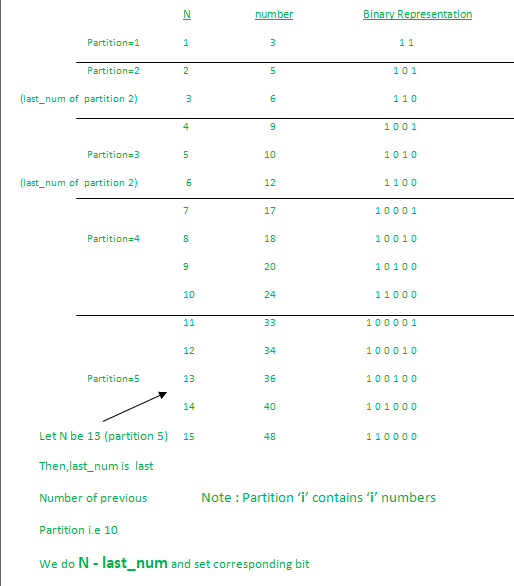# Program to find the Nth natural number with exactly two bits set

Given an integer N, the task is to find the Nth natural number with exactly two bits set.

Examples:

Input: N = 4
Output: 9
Explanation:
Numbers with exactly two bits set: 3, 5, 6, 9, 10, 12, …
4th number in this is 9

Input: N = 15
Output: 48

## Recommended: Please try your approach on {IDE} first, before moving on to the solution.

Naive Approach:

1. Run a loop through all natural numbers, and for each number, check if it has two bits set or not by counting set bits in a number.
2. Print the Nth number having two set bits.

Efficient Approach:

1. Find the leftmost set bit by finding the partition to which N belongs (Partition ‘i’ has ‘i’ numbers in it).
2. To find the other set bit, we’ll have to first find the distance of N from the last number of the previous partition. Based on their difference, we set the corresponding bit.`k = k | (1<<(i))`

Below is the implementation of the above approach:

## C++

 `// C++ Code to  find the Nth number ` `// with exactly two bits set ` ` `  `#include ` `using` `namespace` `std; ` ` `  `// Function to find the Nth number ` `// with exactly two bits set ` `void` `findNthNum(``long` `long` `int` `N) ` `{ ` ` `  `    ``long` `long` `int` `bit_L = 1, last_num = 0; ` ` `  `    ``// Keep incrementing until ` `    ``// we reach the partition of 'N' ` `    ``// stored in bit_L ` `    ``while` `(bit_L * (bit_L + 1) / 2 < N) { ` `        ``last_num = last_num + bit_L; ` `        ``bit_L++; ` `    ``} ` ` `  `    ``// set the rightmost bit ` `    ``// based on bit_R ` `    ``int` `bit_R = N - last_num - 1; ` ` `  `    ``cout << (1 << bit_L) + (1 << bit_R) ` `         ``<< endl; ` `} ` ` `  `// Driver code ` `int` `main() ` `{ ` `    ``long` `long` `int` `N = 13; ` ` `  `    ``findNthNum(N); ` ` `  `    ``return` `0; ` `} `

## Java

 `// Java Code to  find the Nth number ` `// with exactly two bits set ` `class` `GFG{ ` `  `  `// Function to find the Nth number ` `// with exactly two bits set ` `static` `void` `findNthNum(``int` `N) ` `{ ` `  `  `    ``int` `bit_L = ``1``, last_num = ``0``; ` `  `  `    ``// Keep incrementing until ` `    ``// we reach the partition of 'N' ` `    ``// stored in bit_L ` `    ``while` `(bit_L * (bit_L + ``1``) / ``2` `< N) { ` `        ``last_num = last_num + bit_L; ` `        ``bit_L++; ` `    ``} ` `  `  `    ``// set the rightmost bit ` `    ``// based on bit_R ` `    ``int` `bit_R = N - last_num - ``1``; ` `  `  `    ``System.out.print((``1` `<< bit_L) + (``1` `<< bit_R) ` `         ``+``"\n"``); ` `} ` `  `  `// Driver code ` `public` `static` `void` `main(String[] args) ` `{ ` `    ``int` `N = ``13``; ` `  `  `    ``findNthNum(N); ` `} ` `} ` ` `  `// This code is contributed by Princi Singh `

## Python3

 `# Python Code to  find the Nth number ` `# with exactly two bits set ` ` `  ` `  `# Function to find the Nth number ` `# with exactly two bits set ` `def` `findNthNum(N): ` ` `  `    ``bit_L ``=` `1``; ` `    ``last_num ``=` `0``; ` ` `  `    ``# Keep incrementing until ` `    ``# we reach the partition of 'N' ` `    ``# stored in bit_L ` `    ``while` `(bit_L ``*` `(bit_L ``+` `1``) ``/` `2` `< N): ` `        ``last_num ``=` `last_num ``+` `bit_L; ` `        ``bit_L``+``=``1``; ` `     `  ` `  `    ``# set the rightmost bit ` `    ``# based on bit_R ` `    ``bit_R ``=` `N ``-` `last_num ``-` `1``; ` ` `  `    ``print``((``1` `<< bit_L) ``+` `(``1` `<< bit_R)); ` ` `  ` `  `# Driver code ` `if` `__name__ ``=``=` `'__main__'``: ` `    ``N ``=` `13``; ` ` `  `    ``findNthNum(N); ` ` `  ` `  `# This code contributed by PrinciRaj1992 `

## C#

 `// C# Code to  find the Nth number ` `// with exactly two bits set ` `using` `System; ` ` `  `class` `GFG{ ` `   `  `// Function to find the Nth number ` `// with exactly two bits set ` `static` `void` `findNthNum(``int` `N) ` `{ ` `   `  `    ``int` `bit_L = 1, last_num = 0; ` `   `  `    ``// Keep incrementing until ` `    ``// we reach the partition of 'N' ` `    ``// stored in bit_L ` `    ``while` `(bit_L * (bit_L + 1) / 2 < N) { ` `        ``last_num = last_num + bit_L; ` `        ``bit_L++; ` `    ``} ` `   `  `    ``// set the rightmost bit ` `    ``// based on bit_R ` `    ``int` `bit_R = N - last_num - 1; ` `   `  `    ``Console.Write((1 << bit_L) + (1 << bit_R) ` `         ``+``"\n"``); ` `} ` `   `  `// Driver code ` `public` `static` `void` `Main(String[] args) ` `{ ` `    ``int` `N = 13; ` `   `  `    ``findNthNum(N); ` `} ` `} ` ` `  `// This code is contributed by Princi Singh `

Output:

```36
```

Time Complexity : O(Partition of Number)

Attention reader! Don’t stop learning now. Get hold of all the important DSA concepts with the DSA Self Paced Course at a student-friendly price and become industry ready.

My Personal Notes arrow_drop_upCheck out this Author's contributed articles.

If you like GeeksforGeeks and would like to contribute, you can also write an article using contribute.geeksforgeeks.org or mail your article to contribute@geeksforgeeks.org. See your article appearing on the GeeksforGeeks main page and help other Geeks.

Please Improve this article if you find anything incorrect by clicking on the "Improve Article" button below.